Checkout JEE MAINS 2022 Question Paper Analysis : Checkout JEE MAINS 2022 Question Paper Analysis :

# Quantum Theory of Light

## Wave-Particle Duality of Light

Quantum theory describes that matter, and light consists of minute particles that have properties of waves that are associated with them. Light consists of particles known as photons and matter are made up of particles known as protons, electrons, and neutrons. Let’s understand how light behaves as a particle and as a wave.

### Wave Theory of Light

Diffraction is one of the behaviours of waves. Interference is the other behaviour of waves. James Clerk Maxwell showed that light is an electromagnetic wave that travels at the speed of light through space. The light frequency is relevant to its wavelength according to the following relation.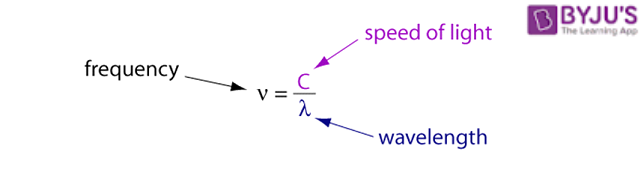Here are the names that were given to regions of the electromagnetic spectrum separately, based on their range of frequency.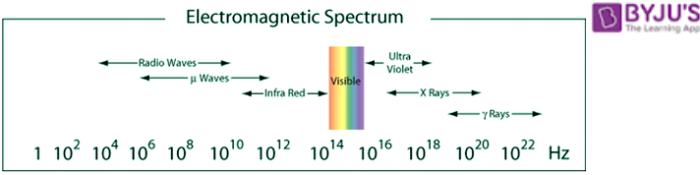### Particle Behaviour of Light

How do we believe that light consists of particles known as photons? This idea is supported by the well-known experiment – The photoelectric effect.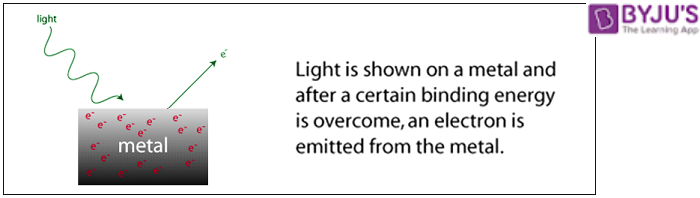The major feature of the photoelectric experiment is that the electron is emitted by the metal with a particular kinetic energy. When you are familiar with the behaviour of waves, you must have also known that a wave is related to its intensity or amplitude. For instance, the bigger the wave in oceans; the higher the energy associated with it. As the light gets brighter, some electrons are emitted while the kinetic energy remains the same.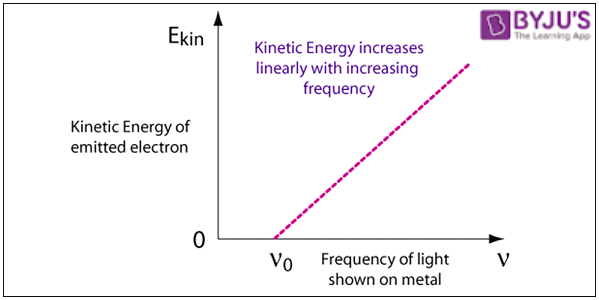There exists a critical frequency for every metal, ν0 lower than which no electrons are not emitted. This describes that the kinetic energy equals the light frequency times a constant, known as Planck’s Constant by the symbol h.

h = 6.63 × 10-34 J · s ← Planck’s Constant

This equation for the kinetic energy of an emitted electron is written as follows.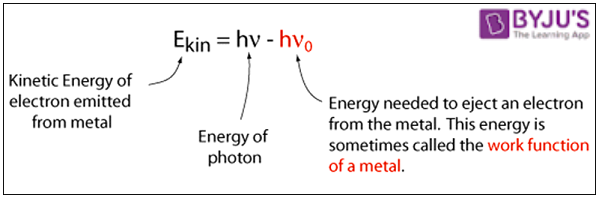The is not consistent when the light is pictured as a wave. A consistent explanation would be when the picture of light comes in discrete packages known as photons, and every photon should have sufficient energy to eject a single electron. Hence, the energy of a single photon is given by,

Ephoton = h ν

Hence, when all the phenomenons are put together, it can be concluded that light is a particle with wave behaviour.

Test your knowledge on Quantum Theory Of Light